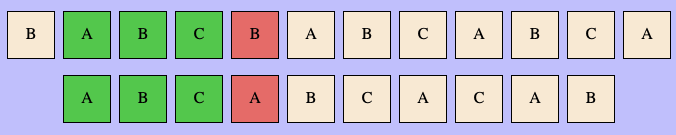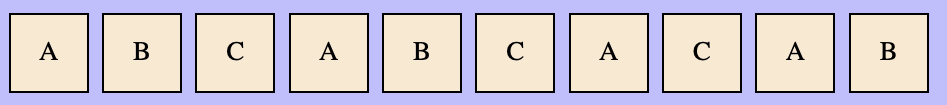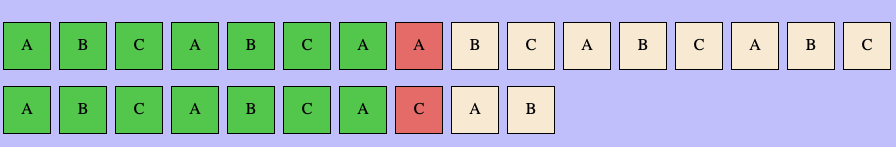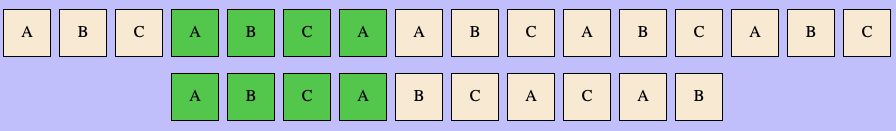# Lecture 21: Pattern Matching, Part II

## Announcement

Final Project, Preliminary Presentations

• Monday, May 1 in class
• includes a substantial component of your project
• demos in small groups
• give/receive constructive feedback on projects

## Last Time

Input.

• a large text, TEXT
• a smaller text, PATTERN

Output.

• “yes” if TEXT contains PATTERN as a substring
• or, starting index of first instance of PATTERN in TEXT
• -1 if PATTERN does not appear

## Naive Pattern Matching

idx = 0;
matches = 0;
while (idx < TEXT.length - PATTERN.length) {
if (matches == PATTERN.length) return idx;
if (TEXT[idx + matches] == PATTERN[matches]) {
matches++;
} else {
idx++;
matches = 0;
}
return (matches == PATTERN.length) ? idx : -1;
}


## Illustration of Procedure

• lec21-naive-pattern-matching.zip

## Credits

• Display: Emi, Megan, Sarah
• Input: Ramisa, Mariam, Sulagna
• Algo: Max, Neville (modified by Will)

## Unforseen Problem

Resetting the state of the algorithm

• after updating text or pattern

Method should be called by update buttons (Input Group), but implementation should be handled by algorithm (Algo Group)

• resetState method

## Search Efficiency

Throughout:

• $n$ is the length of the TEXT
• $m$ is the length of the PATTERN

Last Time, we showed worst-case running time is $\Theta(n \cdot m)$.

• example:
• TEXT = 'aaaaaaaaaaaaa...a'
• PATTERN = 'aaaa....ab'

## Redundant Work

• see illustration of bad example

Question. What redundant/unnecessary work is being done by the algorithm?

## Mis/Matches

What does naive pattern matching do?## A Puzzle

Suppose the following string is matched up to index i = 3, and mismatched at index i = 4. What should our next comparison be?## Another Puzzle

Suppose the following string is matched up to index i = 4, and mismatched at index i = 5. What should be our next comparison be?## Next Move?## Next Move!## A Challenge

Question. Can we perform pattern matching search in such a way that the textIndex never decreases?

• Why should this be possible?
• if we’ve matched characters in TEXT, then we know they are the same as the previous characters in PATTERN
• we can just read these off of the pattern itself
• better yet, we can pre-compute the next offsets for each mis-match

## Establishing Notation• Pattern is $P$, length $m$
• $P_k = P[0..k]$ is the prefix of length $k+1$
• A suffix of length $\ell$ is the last $\ell$ elements of a (sub)pattern

## A Shift Condition?

Question. Suppose we’ve matched $P_{k}$ with our text, but $P[k+1]$ is a mismatch. Under what condition can we match $P_i$ with our text?## A Shift Condition!

Question. Suppose we’ve matched $P_{k}$ with our text, but $P[k+1]$ is a mismatch. Under what condition can we match $P_i$ with our text?

Answer. We can match $P_i$ with the text if $P_i$ is a suffix of $P_k$## The Prefix Function

Definition. Given a pattern $P$ of length $m$, the associated prefix function is an array $\pi$ of length $m$ defined as follows:

• $\pi[k] = i$ if $P_{i-1}$ is the longest prefix of $P$ that is a suffix of $P_k$

## Activity

Write the prefix function of this pattern:## Faster Pattern Matching

Question. Given the prefix function $\pi$, how can we compute matches faster?

Idea.

• Deal with first character mismatches as in naive strategy
• Use matches and $\pi$ to do more efficient shifts:

• if first mis-match at index $k+1$, we know matched up to index $k$
• we know that for $i = \pi(k)$, $A_i$ matches the suffix of $A_k$
• $\implies A_i$ also matches the text
• choose next shift to align $A_i$:
• amount is is additional $k - i$

## Demo Time!

lec21-kmp-pattern-matching

## Running Time

Question. What is the running time of the method?

let matched = 0
for (i from 0 to n - 1):
while matched > 0 and P[matched+1] != T[i]
matched = pi[matched]
if P[matched] == T[i]
matched++
if matched == m
return i


## Amortized Analysis!

Observations.

• the while loop does at most matched iterations
• in order to do k iterations, matched must be incremented k times
• each iteration of the for loop increments k once
• $\implies$ total number of while loop iterations is $\leq$ number of for loop iteration

## Still To Do

Computing $\pi$ of $P$ efficiently

• easy: $O(m^2)$ time
• not as easy: $O(m)$ time
• use dynamic programming (see demo code)